## 5.3.3 Periodic ModelTo get a deeper insight into the mechanisms involved in the angular dependence of hysteresis we have performed micromagnetic simulations considering two different models. In both of them the computational region was discretized in a two-dimensional square mesh of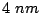single layer units. In our first model, which will be referred as periodic model'', we have considered an infinite array of antidots, corresponding to periodic boundary conditions along X and Y directions and a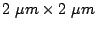modeled region including a circular antidot with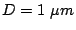. The remanent state was very similar to the saturation in the easy axis direction except for the presence of inhomogeneous moment structures similar to closure domains at the regions of the antidot surfaces (see Fig. 5.16). The thickness of these regions, measured along the average moment direction, was of the order of two times the exchange length of Fe. In the antidot perimeter the magnetization is parallel to the surface except in two small zones where it is parallel to the easy axes. The magnetization at remanence in the center between the antidots is almost directed in the easy axis direction for any applied field angle and the expected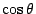behavior is verified for the normalized remanence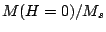as a function of the applied field angle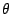.

The angular dependence of the demagnetization is governed by the occurrence of a nucleation-propagation sequence starting from those inhomogeneous magnetic moments structures present at the antidot surfaces and resulting from the magnetostatic energy minimization. The process is shown in Fig. 5.17. The results obtained within this model show the presence of two magnetization jumps (see Fig. 5.18 (a)) for almost all applied field angles (the exceptions are the directions close to the h.a.) and switching field values similar to those observed experimentally. However, the calculated behavior of the angular dependence of the first switching field (see Fig. 5.18(b)) is qualitatively different from that experimentally observed.

Figure 5.18: (a) Hysteresis loops and (b) angular dependence of the switching fields in the antidots arrays simulated in the periodic model in comparison to the experimentally measured values.(a) (b)
2008-04-04